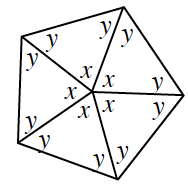### Home > CC1MN > Chapter 10 > Lesson 10.4.1 > Problem10-100

10-100.

Examine the geometric relationships in the diagram at right. Show all of the steps in your solutions for $x$ and $y$.

$x + x + x + x + x = 360º$

$2y + x = 180º$